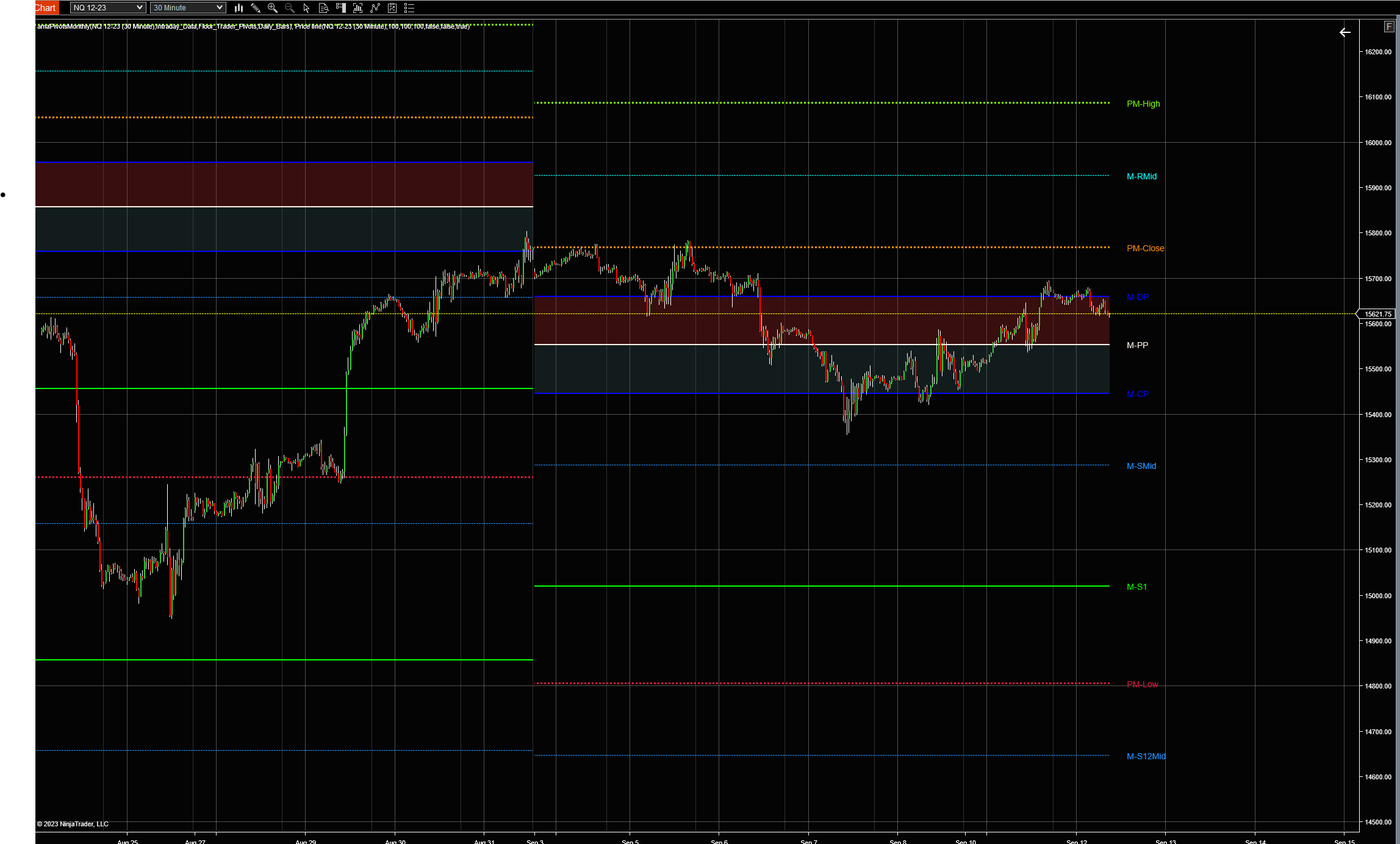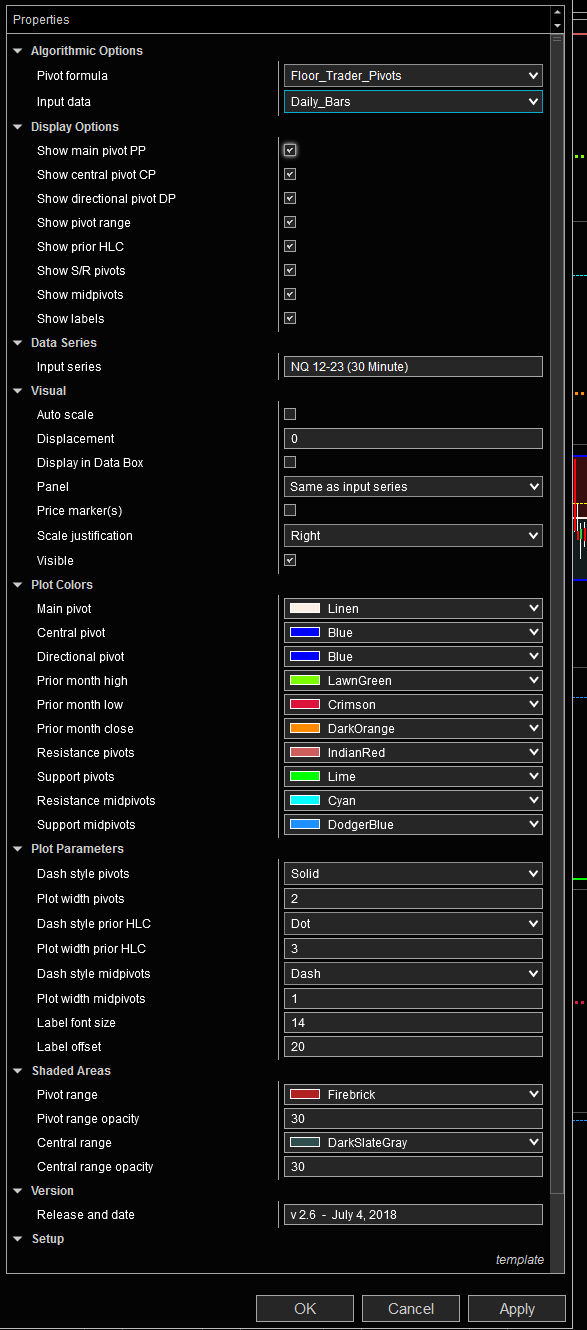# Session Pivots Monthly (amaPivotsMonthly)## The Session Pivots Monthly (amaPivotsMonthly) is an enhanced pivots indicator which allows for displaying floor pivots, wide pivots, Jackson Zones and Fibonacci pivots.

All pivots are calculated from daily bars (default setting) or from the bar series that has been selected as input series. The indicator may be used to display ETH pivots on a full session chart or RTH pivots on a regular session chart. It is not designed to display RTH pivots on a full session chart. When pivots are calculated from daily bars, they are typically calculated from the full session high, the full session low and the settlement price. However, the daily data depends on the data supplier and reflects the daily high, low and close as shown on a daily chart.

Pivot range: The indicator is set to display the pivot range which is the range between CP and DP. The pivot range is a symmetrical range around the main pivot PP. A wide pivot range follows after a trending month with a close near the highs or the lows. A narrow pivot range indicates that the prior month was a balancing month and closed near the central pivot.

Prior month high, low and close: The indicator also displays the high, low and close for the prior trading month. These levels often act as support or resistance during the current trading month. Moreover, prior month high, low and close reflect the input data for calculating all pivot values and can be visually checked against the chart bars, while this is not possible for the pivots themselves.

Pivot formulas:

All options: H = prior month high, L = prior month low, C = prior month close, X = H – L
Main Pivot: P = (H+L+C)/3
Central Pivot: CP = (H+L)/2
Directional Pivot: DP = 2*P – CP
Floor Pivots: P = (H+L+C)/3, R1 = 2*P – L, S1 = 2*P – H, R2 = P + X, S2 = P – X, R3 = R1 + X, S3 = S1 – X, R4 = R3 + (P-L), S4 = S3 – (H-P)
Wide Pivots: P = (H+L+C)/3, R1 = 2*P – L, S1 = 2*P – H, R2 = P + X, S2 = P – X, R3 = R2 + X, S3 = S2 – X, R4 = R3 + X, S4 = S3 – X
Jackson Zones: R1 = P + 0.5*X, S1 = P – 0.5*X, RB1 = P + 0.618*X, SB1 = P – 0.618*X, R2 = P + X, S2 = P – X, RB2 = P + 1.382*X, SB2 = P – 1.382*X
Fibonacci  : R1 = P + 0.382*X, S1 = P – 0.382*X, R2 = P + 0.618*X, S2 = P – 0.618*X, R3 = P + X, S3 = P – X, R4 = P + 1.382*X, S4 = P – 1.382*X